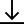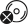# Literacy in Maths - Maths Across the Curriculum

\$24.95

Instant DownloadCD not includedUnderstanding the language of maths is critical to understanding mathematical concepts. This literacy resource explores the use of mathematical concepts and calculations across the subjects of the school curriculum.

Each topic contains a list of words which are featured in a range of literacy activities for students of all ability levels. The activities provide an emphasis on interpreting, analysing, calculating how mathematics is integral to all areas of life.

Topics Include:

• Agriculture - percentage, proportion, area
• Commerce - rates, interest, income
• Dance - time, fractions, compass points
• Food technology - problem solving, volume, capacity, cost
• Geography - scale, grid reference, interpreting graphs
• History - timelines, dimensions
• Metal - percentage, converting units, imperial system
• Photography - capacity, length, number sequences

#### Product Details

SKU:
9781925710076
Year:
Secondary - Junior
Year:
Secondary - Middle
Subject:
Mathematics
Series:
Instant Lessons